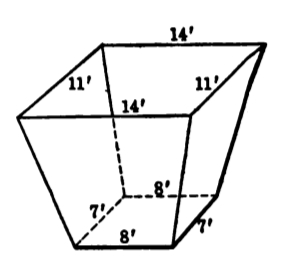# Solid Mensuration: Prismatoid

2 posts / 0 new
RavenBaks
Solid Mensuration: Prismatoid

The altitude of the storage bin shown in the sketch is 12ft and the bases are parallel rectangles having the dimensions indicated. Find the capacity.Tags:
Jhun Vert$A_1 = 8 \times 7 = 56 ~ \text{ft}^2$

$A_2 = 14 \times 11 = 154 ~ \text{ft}^2$

$A_m = \dfrac{8 + 14}{2} \times \dfrac{7 + 11}{2} = 99 ~ \text{ft}^2$

$L = 12 ~ \text{ft}$

$V = \frac{1}{6}L(A_1 + 4A_m + A_2)$

• Mathematics inside the configured delimiters is rendered by MathJax. The default math delimiters are $$...$$ and $...$ for displayed mathematics, and $...$ and $...$ for in-line mathematics.